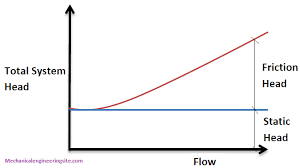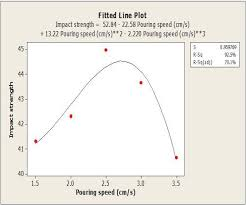## How to Calculate and Solve for Rated Static Head | Design of Gating SystemThe image above represents rated static head.

To compute for rated static head, three essential parameters are needed and these parameters are Height of the Sprue from its Top to the Point of Metal Entry into the Mould Cavity (H), Height of the Casting System from its top (P) and Height of the Casting (C).

The formula for calculating rated static head:

Hs = H – P2 / 2C

Where:

H = Height of the Sprue from its Top to the Point of Metal Entry into the Mould Cavity
P = Height of the Casting from its Top
C = Height of the Casting

Let’s solve an example;
Find the rated static head when the height of the sprue from its top to the point of metal entry into the mould cavity is 9,the height of the casting from its top is 5 and the height of the casting is 11.

This implies that;

H = Height of the Sprue from its Top to the Point of Metal Entry into the Mould Cavity = 9
P = Height of the Casting from its Top = 5
C = Height of the Casting = 11

Hs = H – P2 / 2C
Hs = 9 – 52 / 2 x 11
Hs = 9 – 25 / 22
Hs = 9 – 1.136
Hs = 7.86

Therefore, the rated static head is 7.86 m.

Calculating the Height of the Sprue from its Top to the Point of Metal Entry into the Mould Cavity when the Rated Static Head, Height of the Casting System from its Top and the Height of the Casting is Given.

H = HsP2 / 2C

Where;

H = Height of the Sprue from its Top to the Point of Metal Entry into the Mould Cavity
P = Height of the Casting from its Top
C = Height of the Casting

Let’s solve an example;
Find the height of the sprue when the rated static head is 25, the height of the casting system from its top is 8 and the height of the casting is 4.

This implies that;

Hs = Rated Static Head = 25
P = Height of the Casting from its Top = 8
C = Height of the Casting = 4

H = HsP2 / 2C
H = 25 + 82 / 2 x 4
H = 25 + 64 / 8
H = 25 + 8
H = 33

Therefore, the height of the casting system from its top is 33.

## How to Calculate and Solve for Pouring Speed | Design of Gating SystemThe image above represents pouring speed.

To compute for poring speed, three essential parameters are needed and these parameters are Co-efficient for friction (μ), Acceleration due to Gravity (g) and Rated head static (Hs).

The formula for calculating pouring speed:

v = μ√(2gHs)

Where:

v = Pouring Speed
μ = Co-efficient for Friction
g = Acceleration due to Gravity

Let’s solve an example;
Find the pouring speed when the co-efficient for friction is 14, acceleration due to gravity is 9 and rated static head is 16.

This implies that;

μ = Co-efficient for Friction = 14
g = Acceleration due to Gravity = 9
Hs = Rated Static Head = 16

v = μ√(2gHs)
v = 14 x √(2 x 9 x 16)
v = 14 x √(288)
v = 14 x 16.97
v = 237.58

Therefore, the pouring speed is 237.58 m/s.

Calculating the Co-efficient for Friction when the Pouring Speed, the Acceleration due to Gravity and the Rated Static Head is Given.

μ = v / √(2gHs)

Where;

μ = Co-efficient for Friction
v = Pouring Speed
g = Acceleration due to Gravity

Let’s solve an example;
Find the co-efficient for friction when the pouring speed is 24, the acceleration due to gravity is 9 and the rated static head is 11.

This implies that;

v = Pouring Speed = 24
g = Acceleration due to Gravity = 9
Hs = Rated Static Head = 11

μ = v / √(2gHs)
μ = 24 / √(2 x 9 x 11)
μ = 24 / √198
μ = 24 / 14.07
μ = 1.70

Therefore, the co-efficient for friction is 1.70.